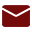## Ü  IF (If condition)

·         It is used to check the condition and if it is true, the next statement in the IF-ENDIF block gets executed.

Example

```
C                   DO        UPCOUNTER
C                   IF        %EOF(CUST)
C     1             SETLL     CUSTR
C                   LEAVE
C                   ENDIF
C                   ENDDO

C                   IF        *IN12=*ON
C                   IF        NODATA1='FALSE' AND NODATA2='FALSE'
C                             AND NODATA3='FALSE' AND I=1
C                   SETON                                        03
C                   ENDIF
C                   ENDIF

C                   IF        %ERROR()
C                   IF        %STATUS(CUST)=1211
C                   OPEN      CUST
C                   ELSEIF    %STATUS(CUST)=1221
C                   EVAL      CSNAME='CCC'
C                   UPDATE(E) CUSTR
C                   ENDIF
C                   ENDIF
```